﻿ Getting Started Tutorials - MechDesigner > Tutorial 14: Gear-Pairs / Rack-Pinions > B: Orbiting Gear Centres > Hypotrochoid: Hypocycloid, Contracted and Protracted

# Hypotrochoid: Hypocycloid, Contracted and Protracted

### B: ORBITING GEAR center

#### Hypocycloid and Hypotrochoid Curves

 Hypotrochoid Curves are produced by a Point an Gear-Pair, with an Internal Mesh. The Gear that Orbits can be Inside or Outside the Stationary Gear.   •Orbiting Inside: The standard Hypocycloid Gears - see below •Orbiting Outside The Peritrochoid Gears - The shape of the Hypotrochoid curve depends on the ratio of the gear-teeth. With gears, the ratio is a rational number. Therefore, the curve will repeat eventually, even if the gear ratio is a ratio of two prime numbers.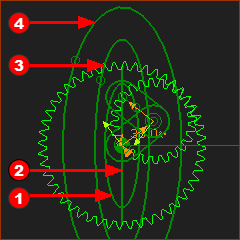Inside The Orbiting Gear is half the diameter of the fixed gear: 1 : 2 Curve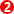is the Hypocycloid It is of the Point on the pitch-circle of the Orbiting Gear - . It is straight line. Hypotrochoid Curves The Hypotrochoid Curves are ellipses. Curves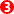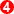are of Point on the outside the pitch-circle . Curveis of a Point  on inside of the pitch-circle.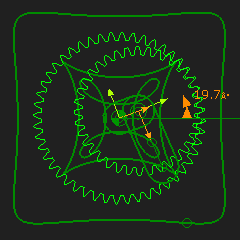The revolving gear inside has 36T:  the outside gear has 48T Hypocycloid The image shows one trace point that is on the pitch-circle It is the Hypocycloid. Hypotrochoid The image shows two curves of points outside the pitch-circle. You can see the curve is near to a square. You can use the Euler-Savary Equation to calculate the best distance from the center of the orbiting gear to give a curve with the best straight-line curve.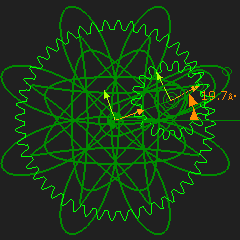Hypocycloid One curve that is given by a point on the pitch-circle - it is the Hypocycloid The Gear Ratio is 48:18 Straight Line Curve with Hypocycloid Gears - Internal Mesh The Video shows the Trace-Point follows a perfectly straight line. You can see that the smaller gear has half the teeth of the other. This is the standard Hypocycloid Straight-Line Epicyclic Gear-Pair configuration.